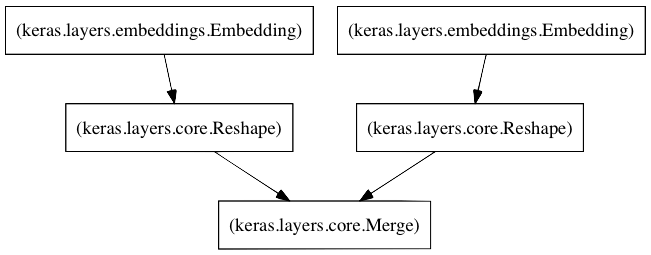# Alkahestmy heroes have always died at the end

## March 7, 2016

### … and logistic regression

Filed under: Technical — cec @ 9:14 pm

A follow-up on the collaborative filter, it occurs to me that it should be possible to add a logistic regression layer in Keras. Â The following is a regularized multinomial logistic regression model:

```from keras.models import Sequential
from keras.layers.core import Dense, Activation
from keras.regularizers import l2

model = Sequential()
init='zero', W_regularizer=l2(0.01)))
model.compile(loss='binary_crossentropy', optimizer='sgd')
```

As with the collaborative filter, you can easily modify the code to use multiple regularizers and different learning algorithms, say Adamax or adam instead of SGD.

Neat.### Collaborative filtering in Keras

Filed under: Technical — cec @ 9:56 am

Ten years ago, Netflix started the Netflix challenge. Â A contest to see if the community could come up with a movie recommendation approach that beat their own by 10%. Â One of the primary modeling techniques that came out of the contest was a set of sparse matrix factoring models whose earliest description can be found atÂ Simon Funk’s website. Â The basic idea is that the actual ratings of movies for each user can be represented by a matrix, say of users on the rows and movies along the columns. Â We don’t have the full rating matrix, instead, we have a very sparse set of entries. Â But if we could factor the rating matrix into two separate matrices, say one that was Users by Latent Factors, and one that was Latent Factors by Movies, then we could find the user’s rating for any movie by taking the dot product of the User row and the Movie column.

One thing that is somewhat frustrating about coding Funk’s approach is that it uses Stochastic Gradient Descent as the learning mechanism and it uses L2 regularization which has to be coded up as well. Â Also, it’s fairly loop-heavy. Â In order to get any sort of performance, you need to implement it in C/C++. Â It would be great if we could use a machine learning framework that already has other learning algorithms, multiple types of regularization, and batch training built in. Â It would also be nice if the framework used Python on the front end, but implemented most of the tight loops in C/C++. Â Sort of like coding the algorithm in Python and compiling with Cython.

My current favorite framework is Keras. Â It uses the Theano tensor library for the heavy lifting which also allows the code to run on a GPU, if available. Â So, here’s a question, can we implement a sparse matrix factoring algorithm in Keras? Â It turns out that we can:

```from keras.layers import Embedding, Reshape, Merge
from keras.models import Sequential,
from keras.callbacks import EarlyStopping, ModelCheckpoint

factors = 20
left = Sequential()
right = Sequential()
model = Sequential()
callbacks = [EarlyStopping('val_loss', patience=2), \
ModelCheckpoint('movie_weights.h5', save_best_only=True)]
model.fit([Users, Movies], Ratings, batch_size=1000000, \
validation_split=.1, callbacks=callbacks)
```

Ta da! Â We’ve just created a left embedding layerÂ that creates a Users by Latent Factors matrix and a right embedding layer that creates a Movies by Latent Factors matrix. Â When the input to these is a user id and a movie id, then they return the latent factor vectors for the user and the movie, respectively. Â The Merge layer then takes the dot product of these two things to return rating. Â We compile the model using MSE as the loss function and the AdaMax learning algorithm (which is superior to Sparse Gradient Descent). Â Our callbacks monitor the validation loss and we save the model weights each time the validation loss has improved.

The really nice thing about this implementation is that we can model hundreds of latent factors quite quickly. Â In my particular case, I can train on nearly 100 million ratings, half a million users and almost 20,000 movies in roughly 5 minutes per epoch, 30 epochs – 2.5 hours. Â But if I use the GPU (GeForce GTX 960), the epoch time decreases to 90s for a total training time of 45 minutes.### Is this thing on?

Filed under: Uncategorized — cec @ 8:51 am

Let’s see, it’s been about 5 (?!) years since I’ve written anything here. Â Since then, I’ve gotten married and nowÂ have a two year old daughter. Â My old company was acquired by a large defense contractor, I left a few months ago and I’m now working on machine learning for a medical device company. Â This space may wind up being used to make machine learning notes to myself.